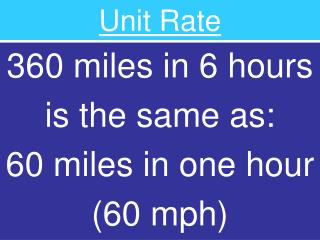DownloadDownload PresentationUnit Rate

# Unit Rate

Télécharger la présentation## Unit Rate

- - - - - - - - - - - - - - - - - - - - - - - - - - - E N D - - - - - - - - - - - - - - - - - - - - - - - - - - -
##### Presentation Transcript

1. Unit Rate 360 miles in 6 hours is the same as: 60 miles in one hour (60 mph)

2. Solving Proportions Cross Multiply: 3 x 4 And divide by the number across the letter 12 / 2 = 6

3. Finding Percents

4. What number is50%of 32 Cross Multiply x=(50x32)/100 = 16

5. What % is16of 32 Cross Multiply x=(16x100)/32=50%

6. 16 is50%of what number? Cross Multiply x=(16x100)/50=32

7. Unit Rate 360 miles in 6 hours is the same as: 60 miles in one hour (60 mph)

8. Solving Proportions Cross Multiply: 3 x 4 And divide by the number across the letter 12 / 2 = 6

9. Finding Percents

10. What number is50%of 32 Cross Multiply x=(50x32)/100 = 16

11. What % is16of 32 Cross Multiply x=(16x100)/32=50%

12. 16 is50%of what number? Cross Multiply x=(16x100)/50=32